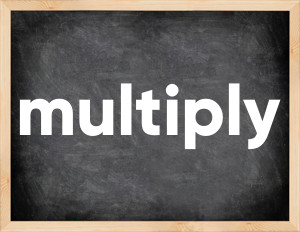# Multiply past tenseThe English verb 'multiply' is pronounced as [ˈmʌltɪplaɪ].
Related to: regular verbs.
3 forms of verb multiply: Infinitive (multiply), Past Simple - (multiplied), Past Participle - (multiplied).

## Here are the past tense forms of the verb multiply

👉 Forms of verb multiply in future and past simple and past participle.
❓ What is the past tense of multiply.

## Multiply: Past, Present, and Participle Forms

Base Form Past Simple Past Participle
multiply [ˈmʌltɪplaɪ]

multiplied [ˈmʌltɪplaɪd]

multiplied [ˈmʌltɪplaɪd]

## What are the 2nd and 3rd forms of the verb multiply?

🎓 What are the past simple, future simple, present perfect, past perfect, and future perfect forms of the base form (infinitive) 'multiply'?

### Learn the three forms of the English verb 'multiply'

• the first form (V1) is 'multiply' used in present simple and future simple tenses.
• the second form (V2) is 'multiplied' used in past simple tense.
• the third form (V3) is 'multiplied' used in present perfect and past perfect tenses.

## What are the past tense and past participle of multiply?

The past tense and past participle of multiply are: multiply in past simple is multiplied, and past participle is multiplied.

### What is the past tense of multiply?

The past tense of the verb "multiply" is "multiplied", and the past participle is "multiplied".

### Verb Tenses

Past simple — multiply in past simple multiplied (V2).
Future simple — multiply in future simple is multiply (will + V1).
Present Perfect — multiply in present perfect tense is multiplied (have/has + V3).
Past Perfect — multiply in past perfect tense is multiplied (had + V3).

### multiply regular or irregular verb?

👉 Is 'multiply' a regular or irregular verb? The verb 'multiply' is regular verb.

## Examples of Verb multiply in Sentences

•   Your investment will multiply in 5 years. (Future Simple)
•   Her money had multiplied sixfold (Past Perfect)
•   You need to multiply three and six (Present Simple)
•   Multiply three numbers and give me the answer (Present Simple)
•   She multiplies these numbers incorrectly (Present Simple)
•   Your fortune will multiply very soon (Future Simple)
•   Поступки преумножаются в результаты (Present Simple)
•   He can't multiply numbers (Present Simple)
•   I will multiply them and name the result (Future Simple)
•   Positive thoughts multiply your happiness (Present Simple)

Along with multiply, words are popular burn down and leave behind.

Verbs by letter: , , , , , , , , , , , , , , , , , , , , , , , , .